# B Parameter Recovery with Reduction from Heuristic

## B.1 Individual Bias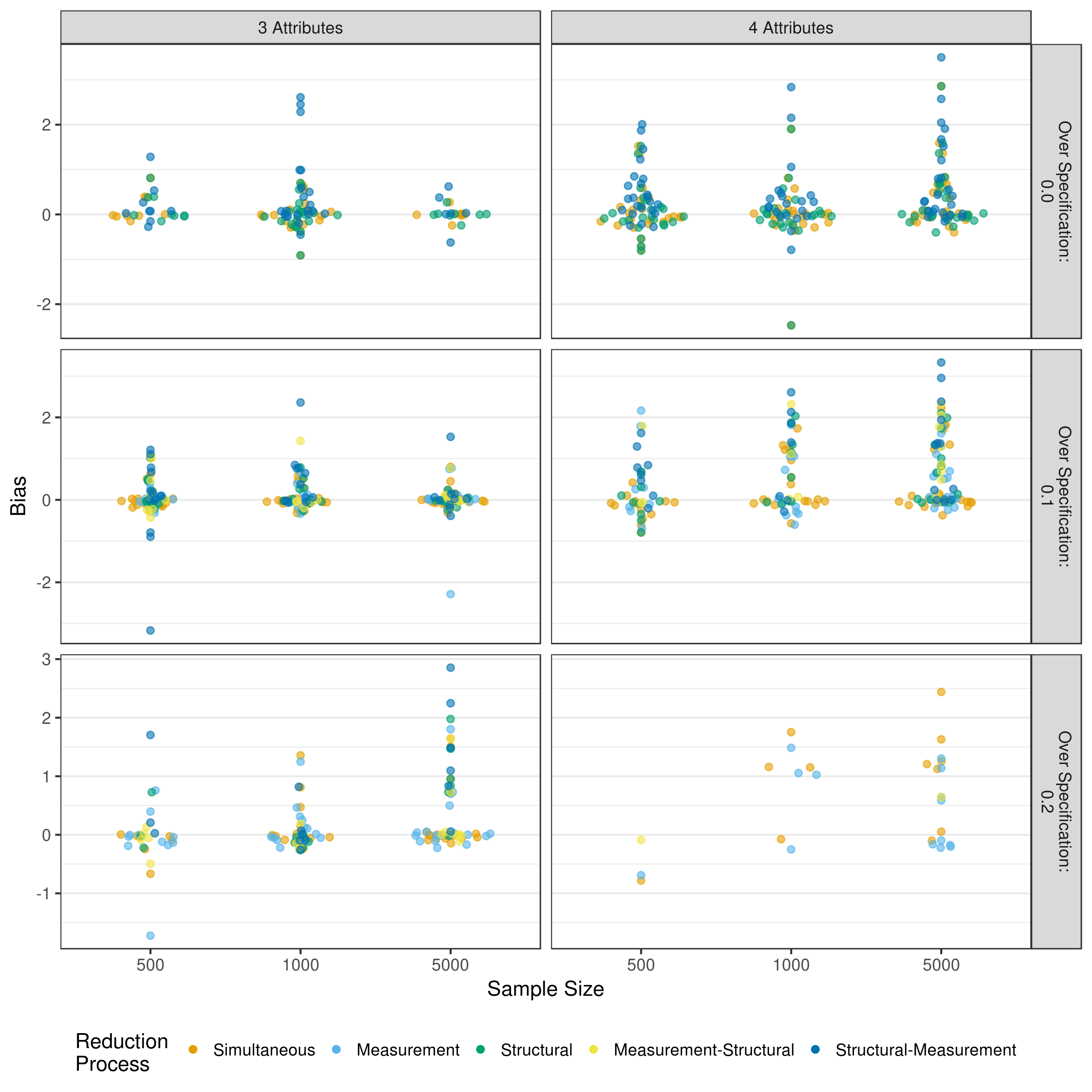Figure B.1: Bias in measurement model intercept estimates when reducing using a heuristic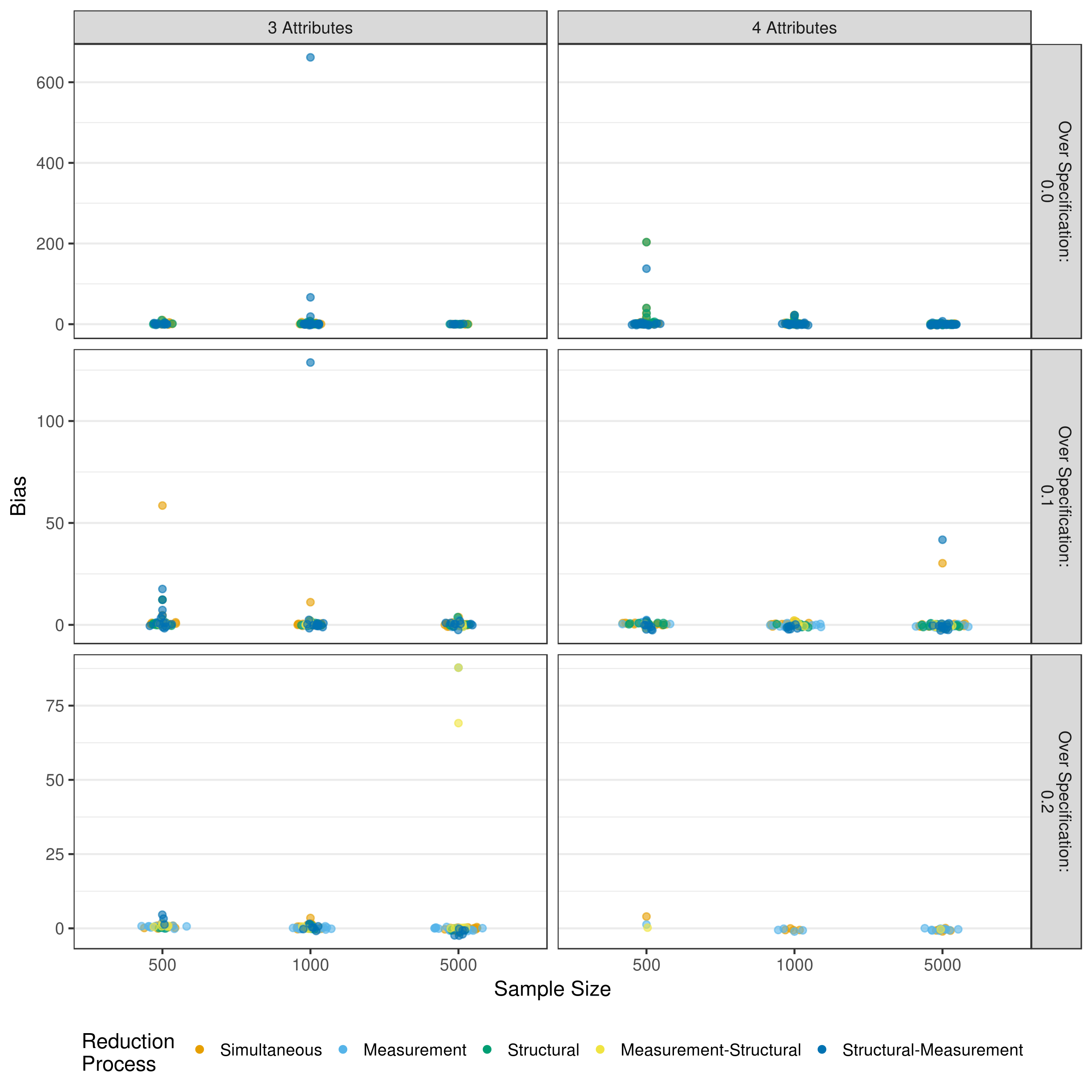Figure B.2: Bias in measurement model main effect estimates when reducing using a heuristic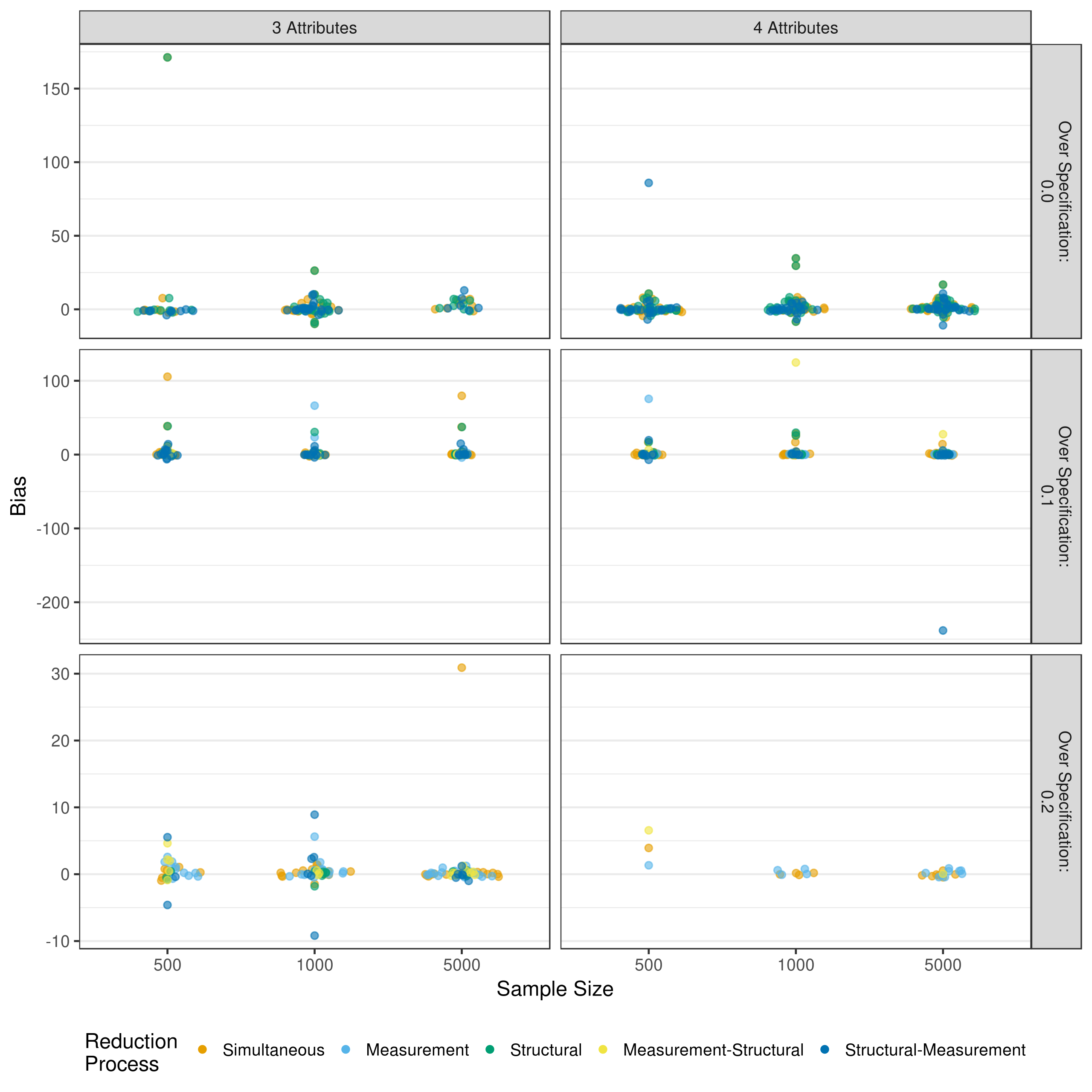Figure B.3: Bias in measurement model 2-way interaction estimates when reducing using a heuristic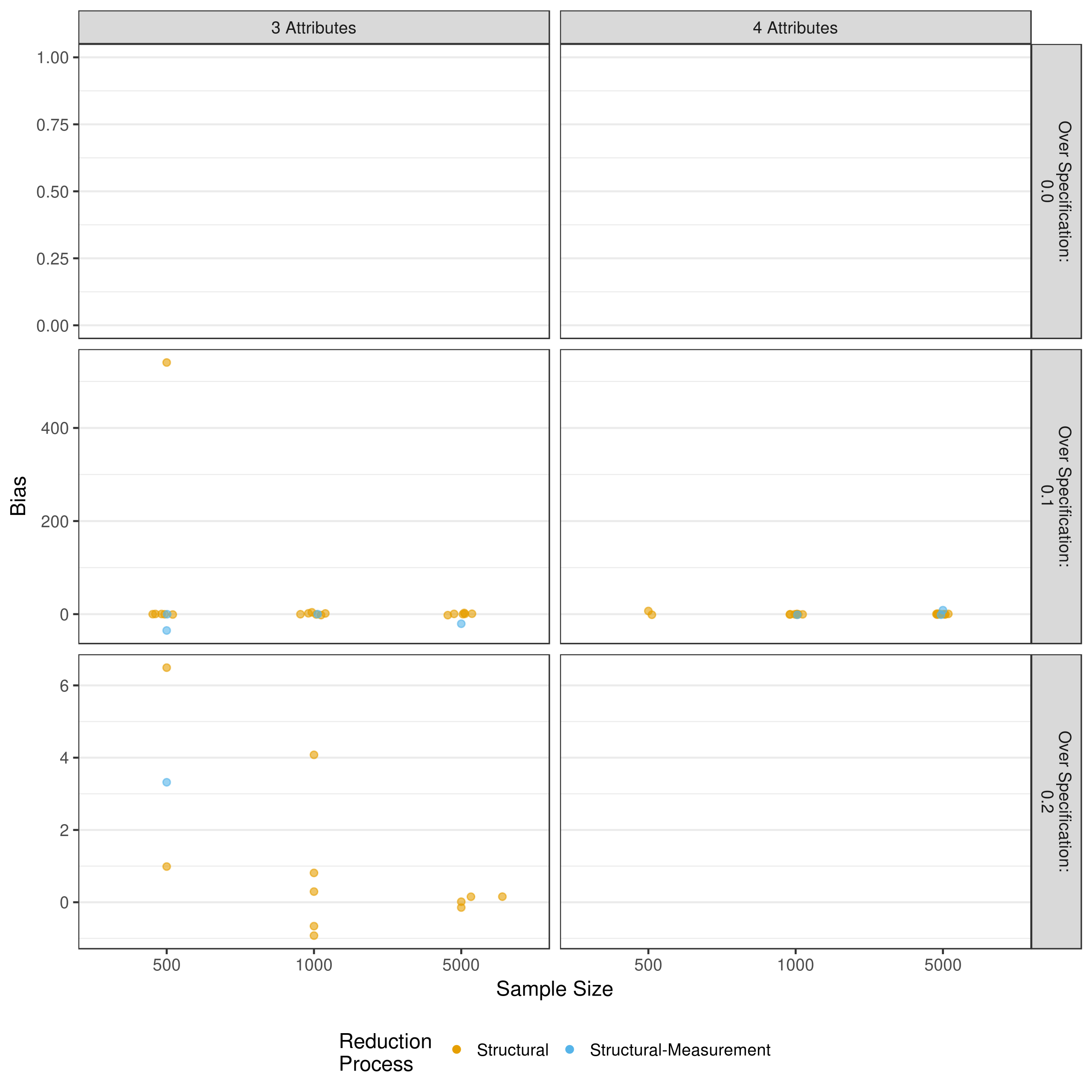Figure B.4: Bias in measurement model 3-way interaction estimates when reducing using a heuristic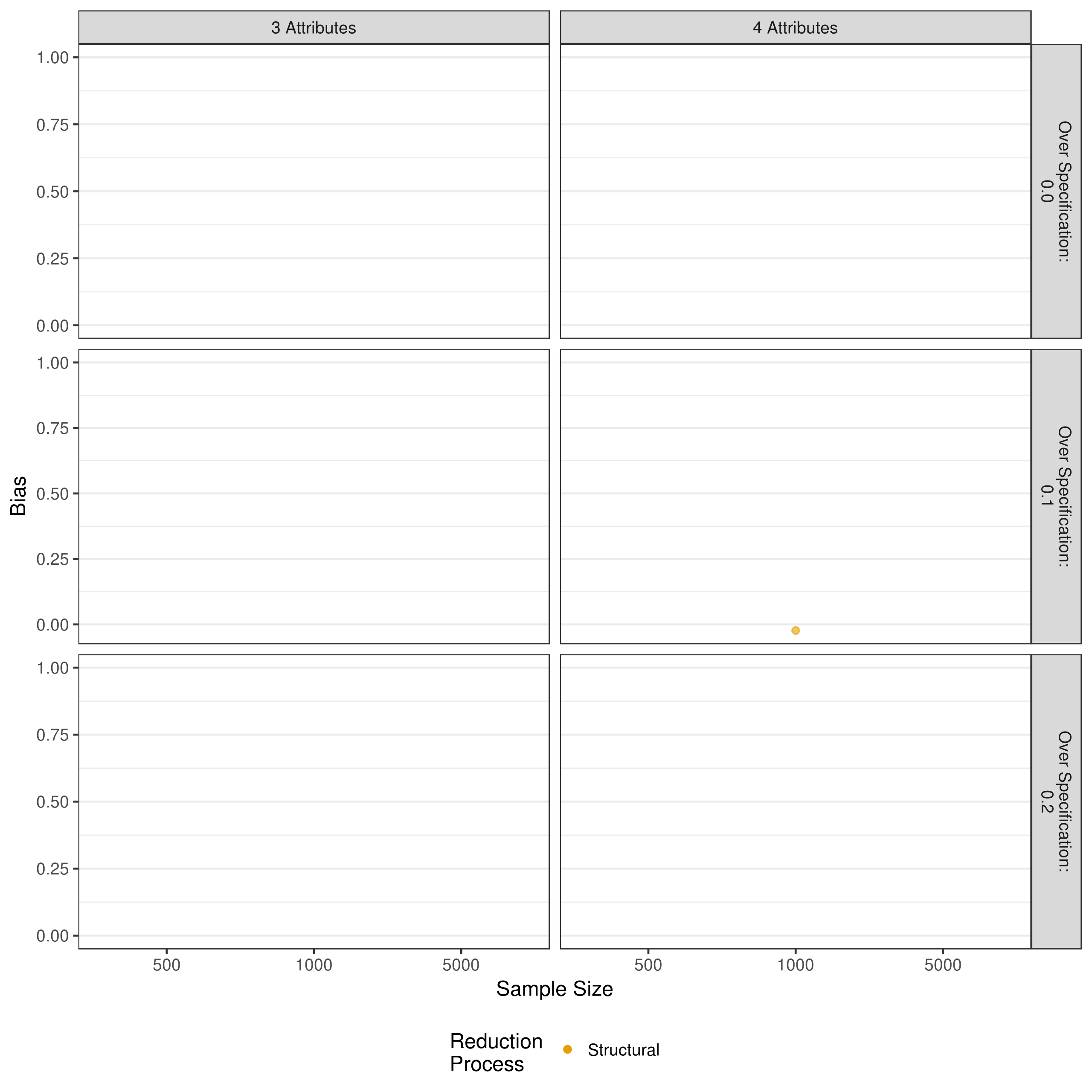Figure B.5: Bias in measurement model 4-way interaction estimates when reducing using a heuristic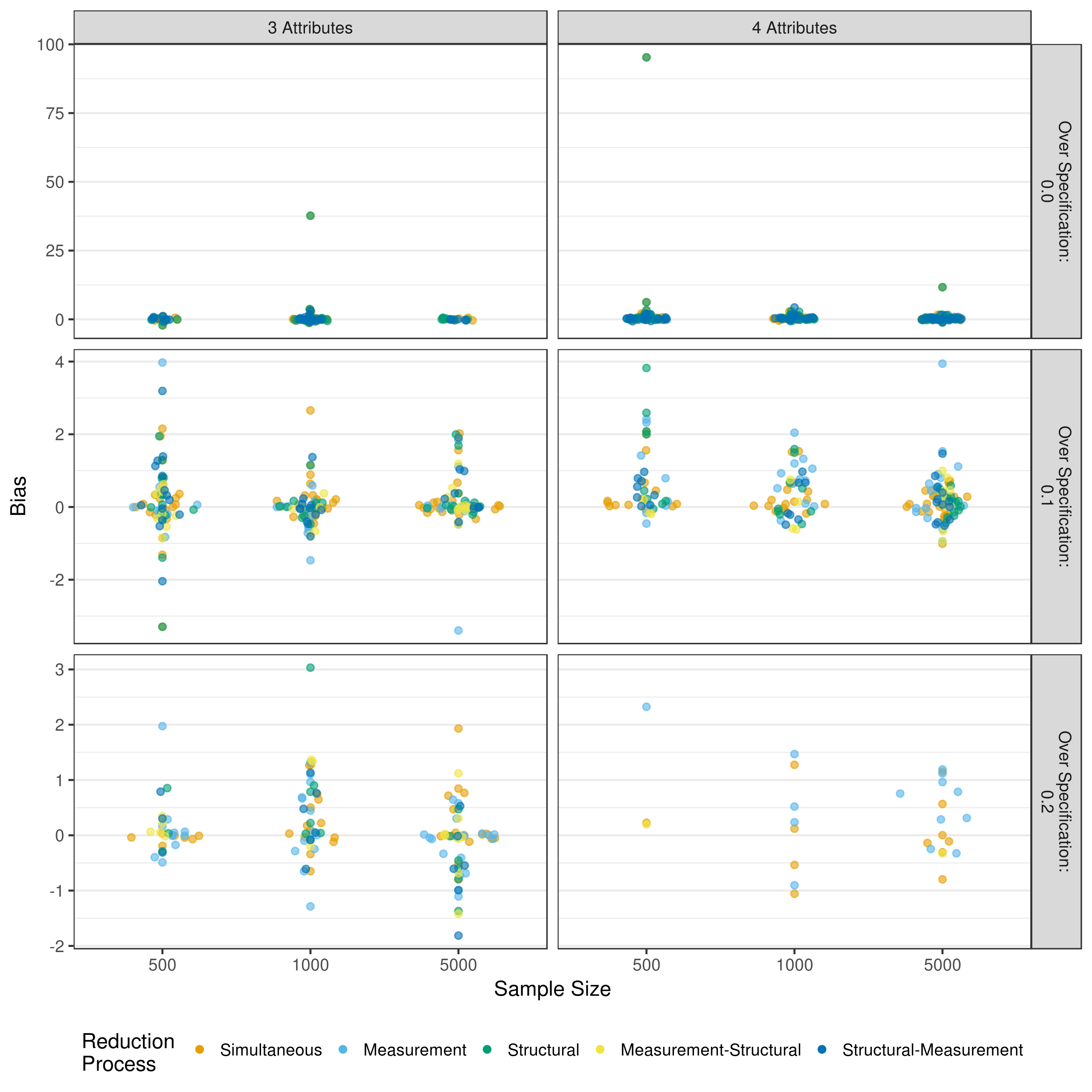Figure B.6: Bias in structural model estimates when reducing using a heuristic

## B.2 Individual Mean Square Error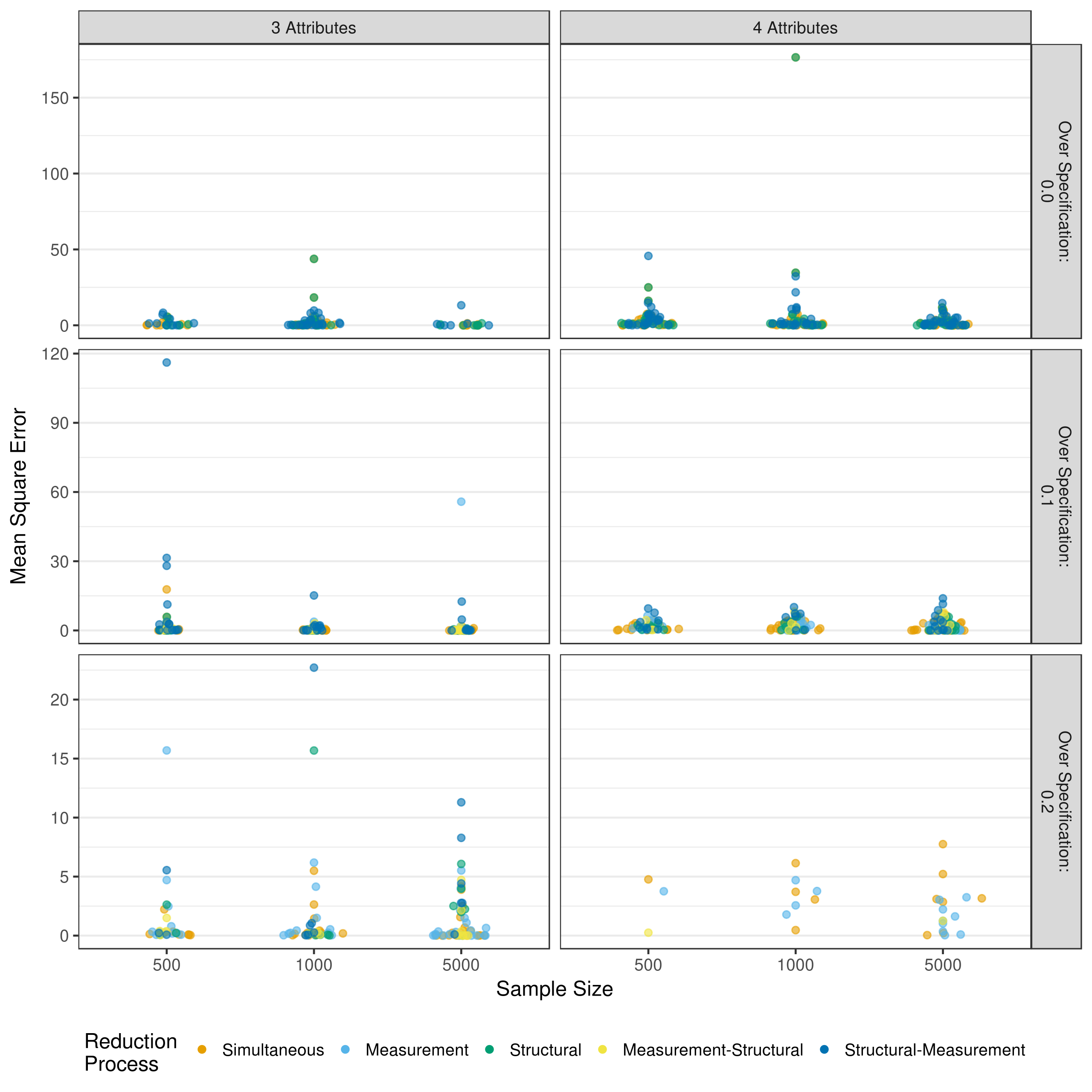Figure B.7: MSE in measurement model intercept estimates when reducing using a heuristic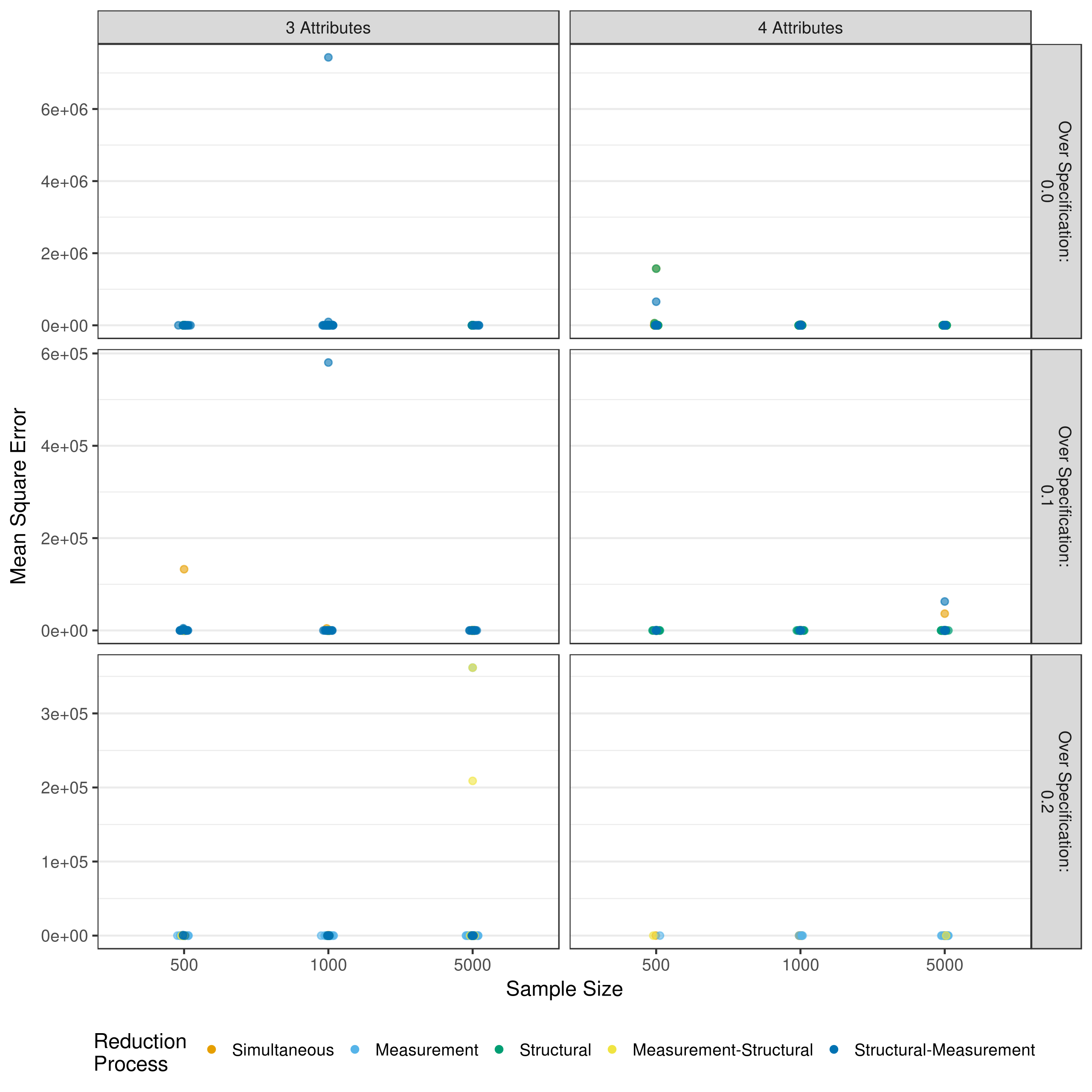Figure B.8: MSE in measurement model main effect estimates when reducing using a heuristic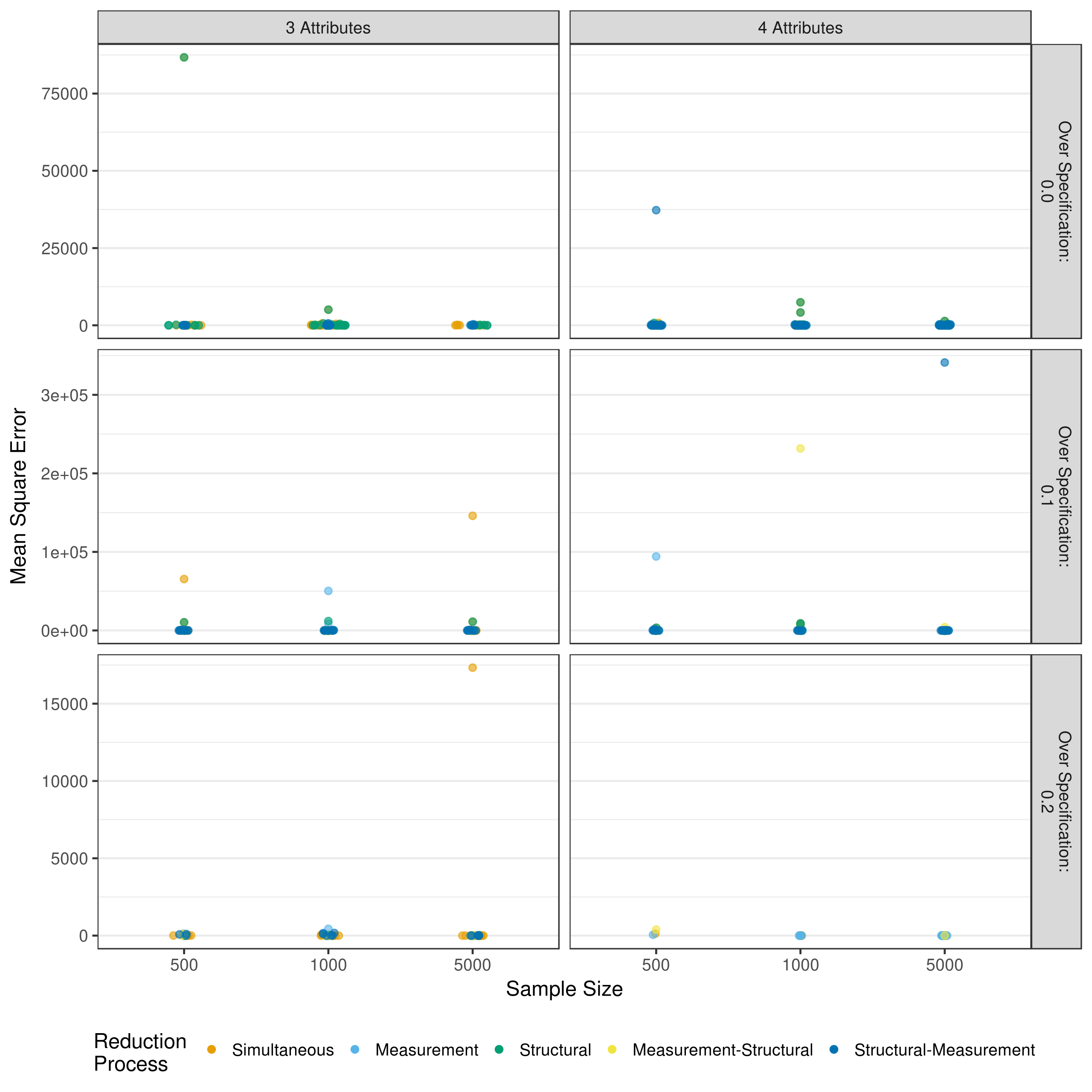Figure B.9: MSE in measurement model 2-way interaction estimates when reducing using a heuristic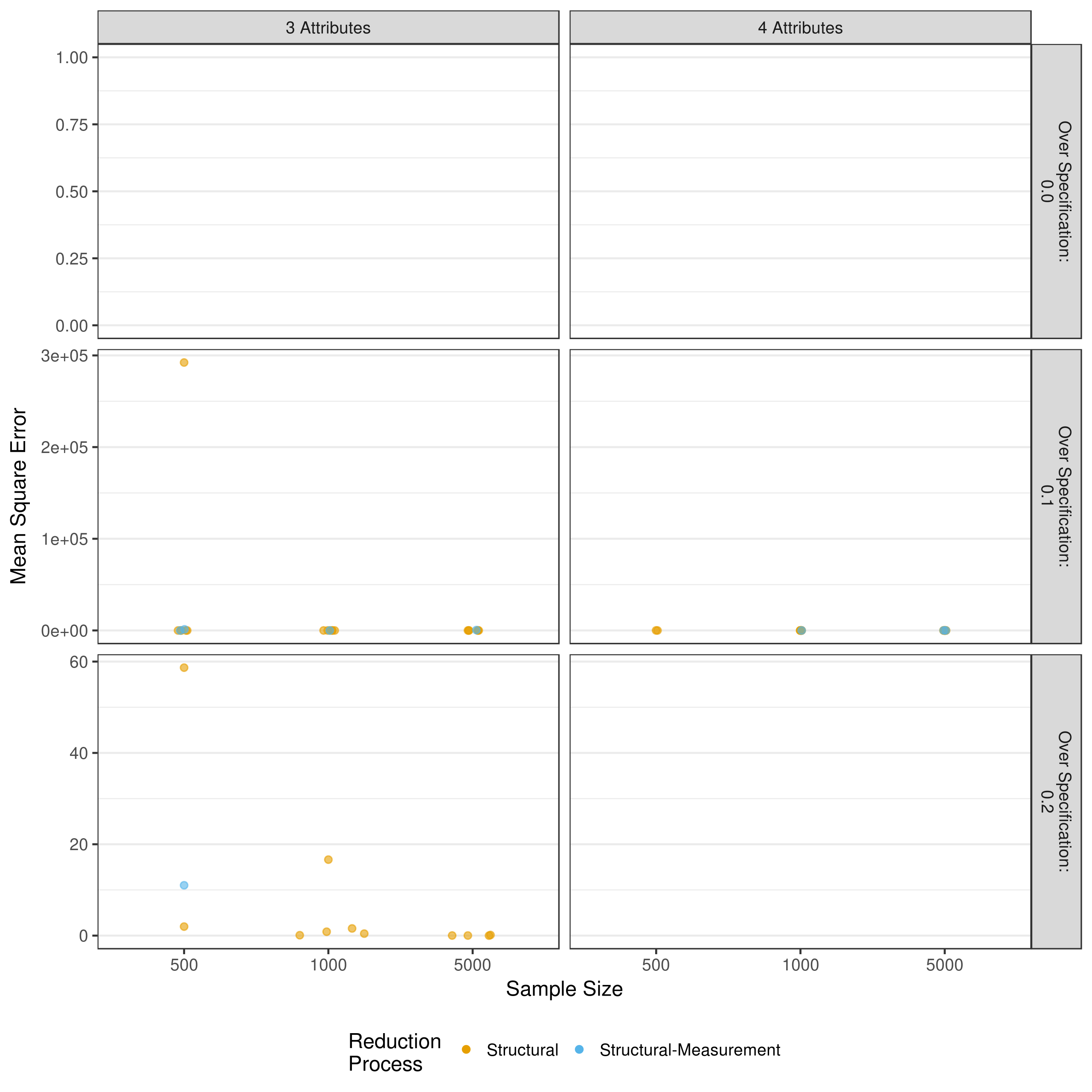Figure B.10: MSE in measurement model 3-way interaction estimates when reducing using a heuristic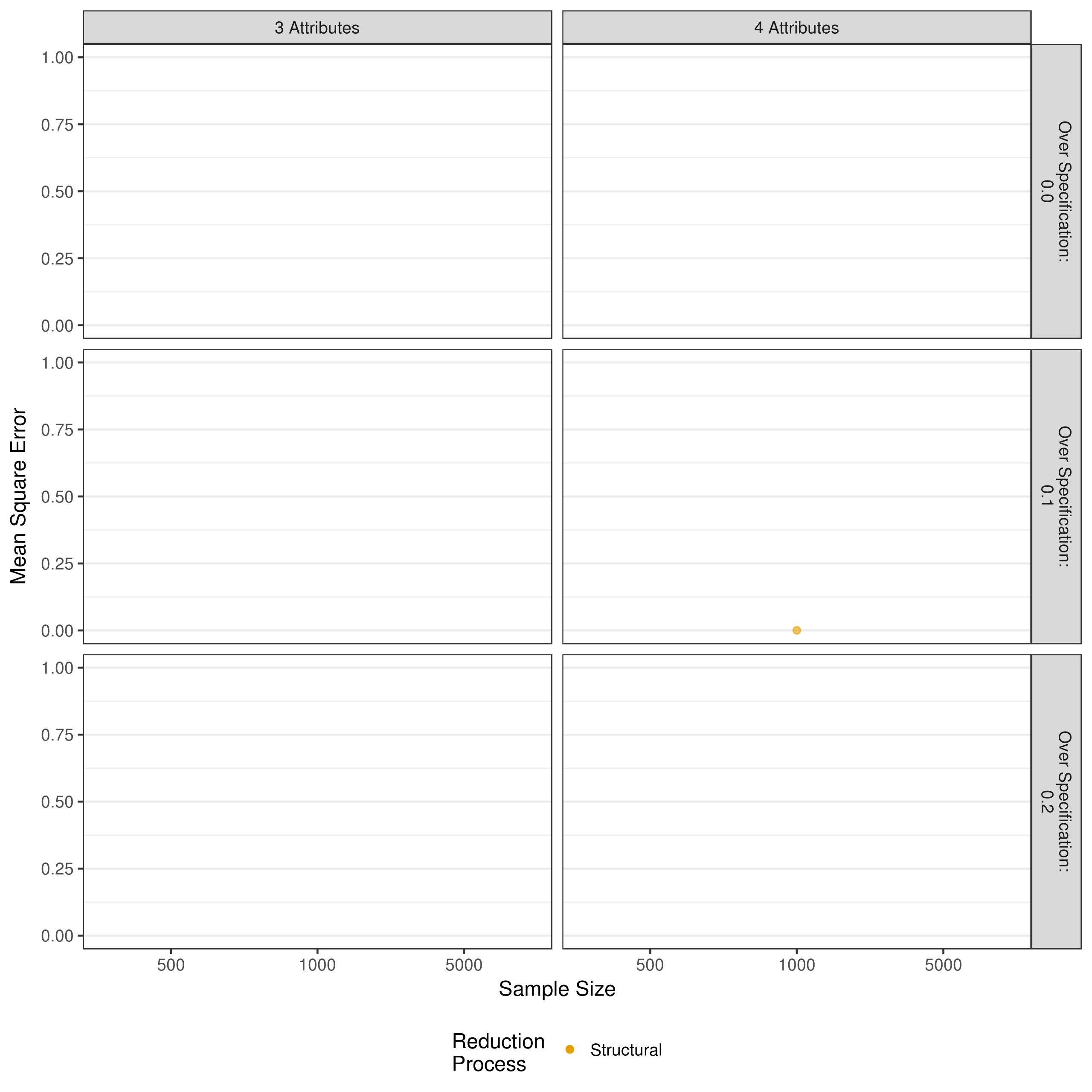Figure B.11: MSE in measurement model 4-way interaction estimates when reducing using a heuristic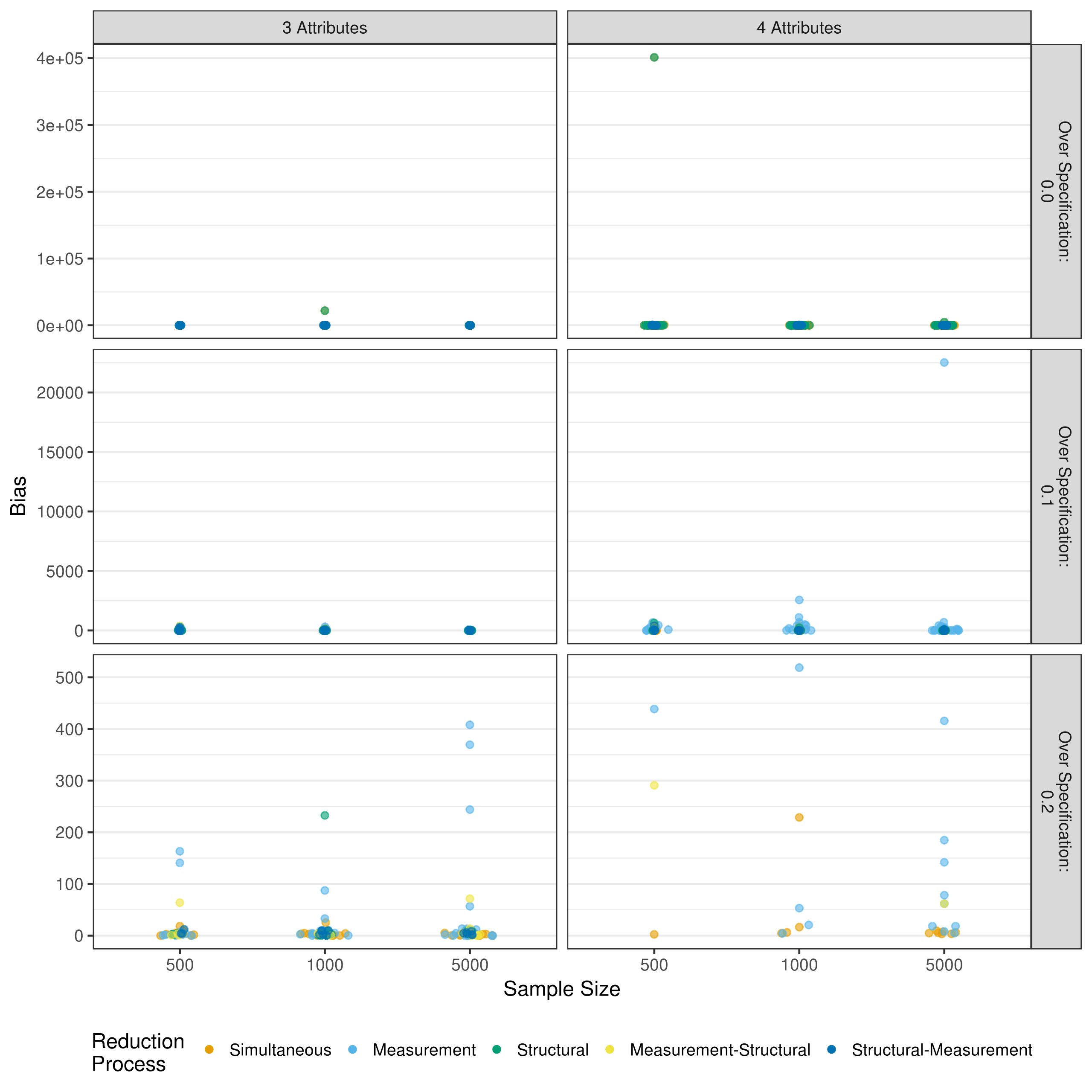Figure B.12: MSE in structural model estimates when reducing using a heuristic